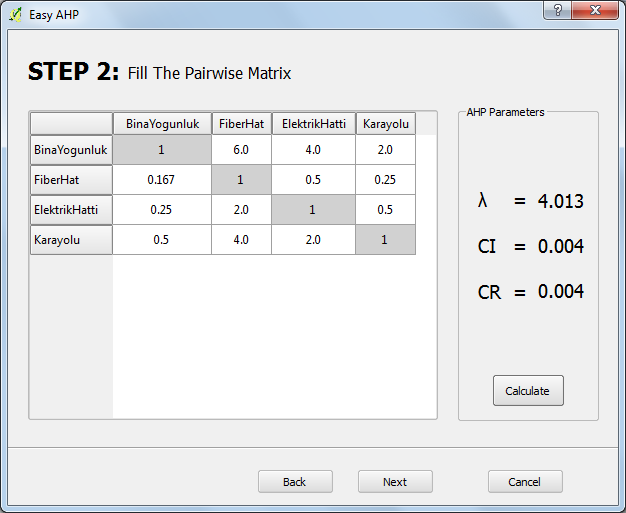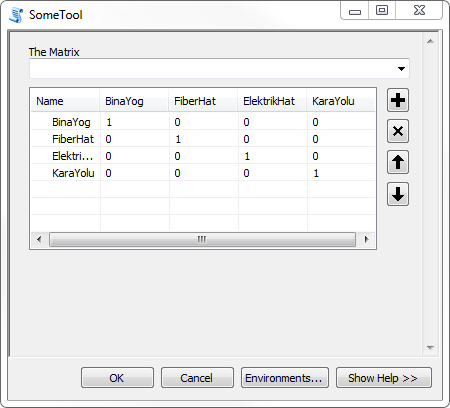# How can I create a matrix as input in Python tool

8397
9
05-14-2016 10:01 AMNew Contributor II

Hi, is there any way to create such matrix:in the input window and fill it with values. Is it possible in Arcpy or anyhow? The only way i can guess is to input excel table but using third-party inputs makes the work-flow more time consuming.

1 Solution

Accepted SolutionsbyMVP Regular Contributor

I discounted a GPValueTable originally, but went back after your post and tried it and seems to do what the OP wants.

```# import modules
import arcpy
import numpy as np

class Toolbox(object):
def __init__(self):
self.label = "SomeToolbox"
self.alias = "SomeToolbox"

# List of tool classes associated with this toolbox
self.tools = [Tool]

class Tool(object):
def __init__(self):
self.label = "SomeTool"
self.description = "SomeTool"
self.canRunInBackground = False

def getParameterInfo(self):
param = arcpy.Parameter(
displayName='The Matrix',
name='TheMatrix',
datatype='GPValueTable',
parameterType="Required",
direction="Input")
param.columns = [['GPString', 'Name'], ['Double', 'BinaYog'], ['Double', 'FiberHat'], ['Double', 'ElektrikHat'], ['Double', 'KaraYolu']]
param.values = [['BinaYog',1,0,0,0],['FiberHat',0,1,0,0],['ElektrikHat',0,0,1,0],['KaraYolu',0,0,0,1]]
return [param]

def execute(self, parameters, messages):
#row[1:] strips off the 1st column
matrix = np.array([row[1:] for row in parameters.values],
dtype=np.float32)EDIT: added code to show how to turn the Value Table into a numpy array.

9 RepliesbyMVP Regular Contributor

There are a ton of ways to do stuff like this in python. Are you trying to have a window where the user can enter values by hand into the matrix? I would guess that the most direct approach would be to just use a script tool.

The input would not come is as matrix but as a list of parameters which you then could add to NumPy matrix if you need to do matrix operations.

Or one could have the user make in as an xls or csv and then have the script tool take that as an input....New Contributor II

I know i can create a list of parameters, im asking if it's possible to use matrix instead. Thanks for showing interest anyway. :)byMVP Esteemed Contributor

No, there is no array input directly, except through numpy, especially if you are lookng to emulate that dialogbyMVP Regular Contributor

Not with a python script tool or toolbox. You could build a custom form with pyside/pyqt and use a QTableWidget for the matrix.byMVP Esteemed Contributor

If you set up a Python toolbox (.pyt) you can dynamically create a value table parameter input. It's fairly complicated but you do have a lot of control, but still within the GP framework so you don't have to custom-build forms with Qt etc.byMVP Regular Contributor

I discounted a GPValueTable originally, but went back after your post and tried it and seems to do what the OP wants.

```# import modules
import arcpy
import numpy as np

class Toolbox(object):
def __init__(self):
self.label = "SomeToolbox"
self.alias = "SomeToolbox"

# List of tool classes associated with this toolbox
self.tools = [Tool]

class Tool(object):
def __init__(self):
self.label = "SomeTool"
self.description = "SomeTool"
self.canRunInBackground = False

def getParameterInfo(self):
param = arcpy.Parameter(
displayName='The Matrix',
name='TheMatrix',
datatype='GPValueTable',
parameterType="Required",
direction="Input")
param.columns = [['GPString', 'Name'], ['Double', 'BinaYog'], ['Double', 'FiberHat'], ['Double', 'ElektrikHat'], ['Double', 'KaraYolu']]
param.values = [['BinaYog',1,0,0,0],['FiberHat',0,1,0,0],['ElektrikHat',0,0,1,0],['KaraYolu',0,0,0,1]]
return [param]

def execute(self, parameters, messages):
#row[1:] strips off the 1st column
matrix = np.array([row[1:] for row in parameters.values],
dtype=np.float32)EDIT: added code to show how to turn the Value Table into a numpy array.New Contributor II

It does the job, but anyway it is some kind of trick, isn't it? :DbyMVP Regular Contributor

It's the closest you'll get to a proper matrix/spreadsheet in the ArcPy/geoprocessing framework. Otherwise you'll have to use an external GUI toolkit, like Qt.

What do you mean "some kind of trick"?byMVP Esteemed Contributor

Of course the options begs the questions as to where in the workflow does this matrix need to be created? Is it a need that this step be calculated within the process or can the process not be broken down into a series of steps... some requiring arcmap be open and running and others not.  This information will probably be useful in deciding on the complexity of what you are going to have to do to ensure everything fits together.